# The Cot-X Family of Distributions with Application

Department of Biostatistics, USA

Received Date: August 18, 2019  Published Date: August 20, 2019

#### Abstract

We modify the cotangent function into a new statistical distribution and show its applicability.

Keywords: Trigonometric functions; Statistical distributions; Breast cancer; Odds T-X Contents

#### Introduction

Statistical distributions arising from trigonometric functions have populated the literature, and for example, see [1-4]. On the other hand, the T-X(W) family of distributions appeared in , and in the special case the random variable T has support [0, ∞), and the weight function is given bywe get the so-called Odds T-X family of distributions with the following integral representation for its CDF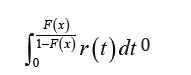where the random variable X has CDF F (x) and the random variable T has PDF

By these observations, this paper unfolds as follows. We introduce a so-called Cot-X family of distributions in Section 2, and in Section 3 we introduce a so called Cot Odds T-X family of distributions. Section 4 and Section 5, show applicability of the new families, and the last section is devoted to the conclusions.

#### The New Family

The CDF of Cot-X is defined as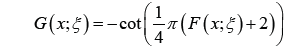where x ∈ R, the baseline distribution has CDF F (x; ξ) and PDF f(x; ξ). By differentiat-ing the CDF, the PDF of Cot-X is given as#### A New Variant of the Odds T-X Family of Distributions

Let T be a random variable with support [0, ∞), whose PDF and CDF are given by r(t; ξ) and R(t; ξ), respectively, with ξ being a vector of parameters in the distribution of T , and let X be a random variable with PDF f(x; β) and CDF F (x; β), where β is a vector of parameters in the distribution of X. We define the Cot Odds T-X family of distributions with the following integral for its CDF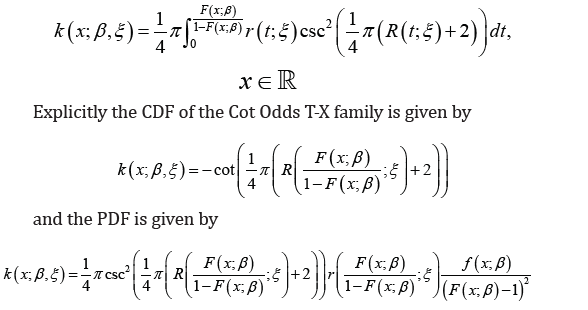#### Practical Illustration of Cot-X

We assume X is a Dagum random variable with CDFfor x, a, b, c > 0. Now from Section 2 we have the following Proposition 6.1. The CDF of Cot-Dagum is given bywhere x, a, b, c > 0

Remark 6.2. The PDF of Cot-Dagum can be obtained by differentiating the CDF. We write S ∼ CD(a, b, c), if S is a Cot-Dagum random variable (Figure 1).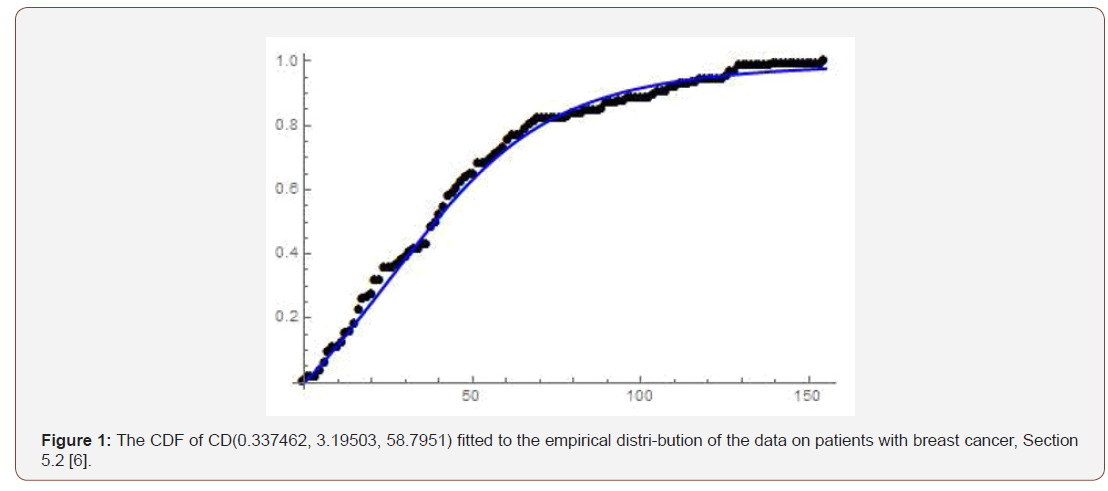#### Practical Illustration of Cot Odds T-X

We assume T is a Frechet random variable with CDFfor x, c, d > 0. We also assume that X is a Weibull random variable with CDF given byfor x, a, b > 0. Now from Section 3, we have the following

Proposition 7.1. The CDF of Cot Odds Frechet-Weibull is given by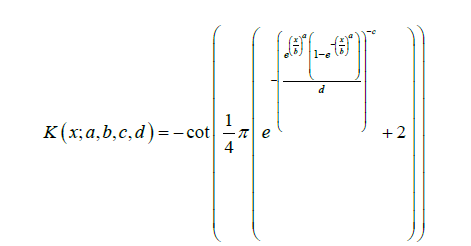Remark 7.2. The PDF of Cot Odds Frechet-Weibull can be obtained by differentiating the CDF. We write J ∼ COF W (a, b, c, d), if J is a Cot Odds Frechet-Weibull random variable (Figure 2).

#### Concluding Remarks

In the present paper we introduced the Cot-X and Cot Odds T-X family of distributions respectively and have shown fit to real-life data. The future interesting problem is to investigate some properties and application of these new classes of statistical distributions.

None.

#### Conflict of Interest

No conflict of interest.

Article Details
Citation
Keywords
Scroll to
Scroll to Top